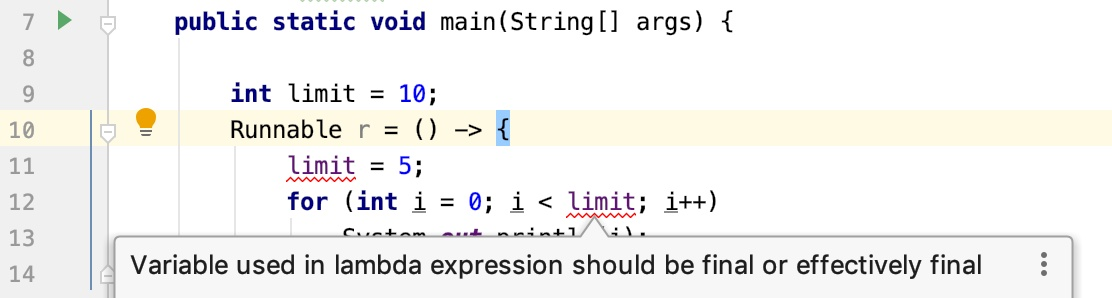# 深入浅出Java 8 Lambda表达式

2022年3月15日
• Java核心
• Java新特性## # 01、初识 Lambda

Lambda 表达式描述了一个代码块（或者叫匿名方法），可以将其作为参数传递给构造方法或者普通方法以便后续执行。考虑下面这段代码：

``````() -> System.out.println("沉默王二")
``````

``````@FunctionalInterface
public interface Runnable
{
public abstract void run();
}
``````

Runnable 接口非常简单，仅有一个抽象方法 `run()`；细心的同学会发现一个陌生的注解 `@FunctionalInterface`，这个注解是什么意思呢？

Note that instances of functional interfaces can be created with lambda expressions, method references, or constructor references.

``````public class LamadaTest {
public static void main(String[] args) {
@Override
public void run() {
System.out.println("沉默王二");
}
}).start();
}
}
``````

``````public class LamadaTest {
public static void main(String[] args) {
}
}
``````## # 02、Lambda 语法

``````( parameter-list ) -> { expression-or-statements }
``````

`()` 中的 `parameter-list` 是以逗号分隔的参数。你可以指定参数的类型，也可以不指定（编译器会根据上下文进行推断）。Java 11 后，还可以使用 `var` 关键字作为参数类型，有点 JavaScript 的味道。

`->` 相当于 Lambda 的标识符，就好像见到圣旨就见到了皇上。

`{}` 中的 `expression-or-statements` 为 Lambda 的主体，可以是一行语句，也可以多行。

1）为变量赋值，示例如下：

``````Runnable r = () -> { System.out.println("沉默王二"); };
r.run();
``````

2）作为 return 结果，示例如下：

``````static FileFilter getFilter(String ext)
{
return (pathname) -> pathname.toString().endsWith(ext);
}
``````

3）作为数组元素，示例如下：

``````final PathMatcher matchers[] =
{
(path) -> path.toString().endsWith("txt"),
(path) -> path.toString().endsWith("java")
};
``````

4）作为普通方法或者构造方法的参数，示例如下：

``````new Thread(() -> System.out.println("沉默王二")).start();
``````

``````public static void main(String[] args) {

int limit = 10;
Runnable r = () -> {
int limit = 5;
for (int i = 0; i < limit; i++)
System.out.println(i);
};
}
``````这个问题发生的原因是因为 Java 规范中是这样规定的：

Any local variable, formal parameter, or exception parameter used but not declared in a lambda expression must either be declared final or be effectively final (§4.12.4)open in new window, or a compile-time error occurs where the use is attempted.

``````final int a;
a = 1;
// a = 2;
// 由于 a 是 final 的，所以不能被重新赋值

int b;
b = 1;
// b 此后再未更改
// b 就是 effectively final

int c;
c = 1;
// c 先被赋值为 1，随后又被重新赋值为 2
c = 2;
// c 就不是 effectively final
``````

1）把 limit 变量声明为 static。

2）把 limit 变量声明为 AtomicInteger。

3）使用数组。

### # 01）把 limit 变量声明为 static

``````public class ModifyVariable2StaticInsideLambda {
static int limit = 10;
public static void main(String[] args) {
Runnable r = () -> {
limit = 5;
for (int i = 0; i < limit; i++) {
System.out.println(i);
}
};
}
}
``````

``````0
1
2
3
4
``````

OK，该方案是可行的。

### # 02）把 limit 变量声明为 AtomicInteger

AtomicInteger 可以确保 int 值的修改是原子性的，可以使用 `set()` 方法设置一个新的 int 值，`get()` 方法获取当前的 int 值。

``````public class ModifyVariable2AtomicInsideLambda {
public static void main(String[] args) {
final AtomicInteger limit = new AtomicInteger(10);
Runnable r = () -> {
limit.set(5);
for (int i = 0; i < limit.get(); i++) {
System.out.println(i);
}
};
}
}
``````

``````0
1
2
3
4
``````

OK，该方案也是可行的。

### # 03）使用数组

``````public class ModifyVariable2ArrayInsideLambda {
public static void main(String[] args) {
final int [] limits = {10};
Runnable r = () -> {
limits = 5;
for (int i = 0; i < limits; i++) {
System.out.println(i);
}
};
}
}
``````

``````0
1
2
3
4
``````

OK，该方案也是可行的。

## # 03、Lambda 和 this 关键字

Lambda 表达式并不会引入新的作用域，这一点和匿名内部类是不同的。也就是说，Lambda 表达式主体内使用的 this 关键字和其所在的类实例相同。

``````public class LamadaTest {
public static void main(String[] args) {
}

public void work() {
System.out.printf("this = %s%n", this);

Runnable r = new Runnable()
{
@Override
public void run()
{
System.out.printf("this = %s%n", this);
}
};
new Thread(() -> System.out.printf("this = %s%n", this)).start();
}
}
``````

Tips：`%s` 代表当前位置输出字符串，`%n` 代表换行符，也可以使用 `\n` 代替，但 `%n` 是跨平台的。

`work()` 方法中的代码可以分为 3 个部分：

1）单独的 this 关键字

``````System.out.printf("this = %s%n", this);
``````

2）匿名内部类中的 this 关键字

``````Runnable r = new Runnable()
{
@Override
public void run()
{
System.out.printf("this = %s%n", this);
}
};
``````

3）Lambda 表达式中的 this 关键字

``````this = com.cmower.java_demo.journal.LamadaTest@3feba861
``````## # 04、最后